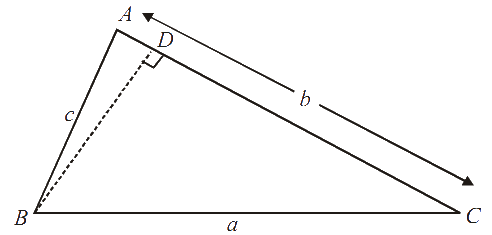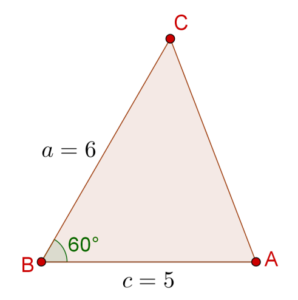# Cosine Law

Cosine Law

## Introduction:

In trigonometry, the law of cosines (also known as the cosine formula or cosine rule) relates the lengths of the sides of a triangle to the cosine of one of its angles.

The law of cosines is useful for computing the third side of a triangle when two sides and their enclosed angle are known, and in computing the angles of a triangle if all three sides are known.

Let's describe is further.

## What is the Cosine Law?

The cosine law states that in any triangle, the following relations will hold:

\begin{align}&{a^2} = {b^2} + {c^2} - 2bc\cos A\\&{b^2} = {c^2} + {a^2} - 2ca\cos B\\&{c^2} = {a^2} + {b^2} - 2ab\cos C\end{align}

✍Note: The Law of Cosines is useful for finding:

• the third side of a triangle when we know two sides and the angle between them.
• the angles of a triangle when we know all three sides.

Proof: We will prove the first of these relations (the others follow similarly).

Consider the following figure:We have:

\begin{align}&{a^2} = B{D^2} + C{D^2}\\&\,\,\,\,\,\, = {\left( {c\sin A} \right)^2} + {\left( {AC - AD} \right)^2}\\&\,\,\,\,\, = {\left( {c\,\sin A} \right)^2} + {\left( {b - c\,\cos A} \right)^2}\\&\,\,\,\,\, = c\, { \sin ^2}A + {b^2} + c \,{ \cos ^2}A - 2bc\cos A\\&\,\,\,\,\, = {b^2} + \left( {c \,{{ \sin }^2}A + c\, {{ \cos }^2}A} \right) - 2bc\cos A\\&\,\,\,\,\, \end{align}

$\Rightarrow \boxed{{a^2} = {b^2} + {c^2} - 2bc\cos A}$

We have proven the cosine law for an acute-angled triangle.Challenge 1: Can you prove that this law holds for obtuse-angled triangles as well?

✍Note: If $$\Delta ABC$$ is a right triangle, right-angled at $$A$$, then the cosine law reduces to the Pythagoras Theorem, since $$\cos A = 0$$ :

${a^2} = {b^2} + {c^2}$

## Solved Examples:

Example 1: In $$\Delta ABC$$, $$AB = 5{\text{ }}cm$$, $$BC = 6{\text{ }}cm$$ and $$\angle B = {60^0}$$. Find the length of $$AC$$.

Solution: Consider the following figure:By cosine law, we have:

\begin{align} {b^2} &= {c^2} + {a^2} - 2ca\cos B \hfill \\ &= 25 + 36 - 2\left( 5 \right)\left( 6 \right)\left( {\frac{1}{2}} \right) \hfill \\ &= 31 \hfill \\ \end{align}

$\Rightarrow \boxed{b = \sqrt {31} }$

Example 2: Let $$s$$ be the semi-perimeter of a triangle:

$s = \frac{{a + b + c}}{2}$

Show that

\begin{align}&\sin \frac{A}{2} = \sqrt {\frac{{\left( {s - b} \right)\left( {s - c} \right)}}{{bc}}} \\&\cos \frac{A}{2} = \sqrt {\frac{{s\left( {s - a} \right)}}{{bc}}} \\&\tan \frac{A}{2} = \sqrt {\frac{{\left( {s - b} \right)\left( {s - c} \right)}}{{s\left( {s - a} \right)}}} \end{align}

Solution: We know the following half-angle relation:

${\sin ^2}\frac{A}{2} = \frac{{1 - \cos A}}{2}$

Next, we make use of the cosine law:

\begin{align}&{\sin ^2}\frac{A}{2} = \frac{{1 - \cos A}}{2}\\&\qquad \quad= \frac{1}{2}\left( {1 - \frac{{{b^2} + {c^2} - {a^2}}}{{2bc}}} \right)\\&\qquad \quad = \frac{1}{2}\left( {\frac{{2bc - {b^2} - {c^2} + {a^2}}}{{2bc}}} \right)\\&\qquad \quad = \frac{1}{2}\left( {\frac{{{a^2} - {{\left( {b - c} \right)}^2}}}{{2bc}}} \right) & ...\left( i \right)\end{align}

Now, we note that:

\begin{align}&{a^2} - {\left( {b - c} \right)^2} = \left( {a + b - c} \right)\left( {a - b + c} \right)\\ &\qquad\qquad\quad\;= \left( {2s - 2c} \right)\left( {2s - 2b} \right)\,\,\,\left( {{\rm{how?}}} \right)\\& \qquad\qquad\quad\;= 4\left( {s - b} \right)\left( {s - c} \right)\end{align}

Using this in (i), we have:

${\sin ^2}\frac{A}{2} = \frac{{\left( {s - b} \right)\left( {s - c} \right)}}{{bc}}$

$\Rightarrow \boxed{\sin \frac{A}{2} = \sqrt {\frac{{\left( {s - b} \right)\left( {s - c} \right)}}{{bc}}} }$

We can prove the second relation similarly:

\begin{align}&{\cos ^2}\frac{A}{2} = \frac{{1 + \cos A}}{2} = \frac{{{{\left( {b + c} \right)}^2} - {a^2}}}{{4bc}}\\&\qquad\quad = \frac{{\left( {b + c + a} \right)\left( {b + c - a} \right)}}{{4bc}}\\&\qquad\quad= \frac{{s\left( {s - a} \right)}}{{bc}}\\ \end{align}

$\Rightarrow \,\boxed{\cos \frac{A}{2} = \sqrt {\frac{{s\left( {s - a} \right)}}{{bc}}} }$

Third relation can be proved by dividing the above two relations:

\begin{align} \tan \frac{A}{2} &= \frac{{\sin \frac{A}{2}}}{{\cos \frac{A}{2}}} \hfill \\ &= \frac{{\sqrt {\frac{{\left( {s - b} \right)\left( {s - c} \right)}}{{bc}}} }}{{\sqrt {\frac{{s\left( {s - a} \right)}}{{bc}}} }} \hfill \\ \end{align}

$\Rightarrow \boxed{\tan \frac{A}{2} = \sqrt {\frac{{\left( {s - b} \right)\left( {s - c} \right)}}{{s\left( {s - a} \right)}}} }$

Example 3: Heron’s Formula: Prove that the area of a triangle in terms of its semi-perimeter and side lengths can be written as:

$\Delta = \sqrt {s\left( {s - a} \right)\left( {s - b} \right)\left( {s - c} \right)}$

Solution: Using the results of example 2 and sine law, we have:

\begin{align}&\Delta = \frac{1}{2}bc\sin A = \frac{1}{2}bc\left( {2\sin \frac{A}{2}\cos \frac{A}{2}} \right)\\&\quad= bc\sin \frac{A}{2}\cos \frac{A}{2}\\&\quad = bc\left( {\sqrt {\frac{{\left( {s - b} \right)\left( {s - c} \right)}}{{bc}}} } \right)\left( {\sqrt {\frac{{s\left( {s - a} \right)}}{{bc}}} } \right)\\&\quad \end{align}

$\Rightarrow \boxed{\Delta = \sqrt {s\left( {s - a} \right)\left( {s - b} \right)\left( {s - c} \right)} }$

Thus, Heron’s Formula can be justified using cosine and sine laws.Challenge 2: In $$\Delta ABC$$, $$AB = 5{\text{ }}cm$$, $$BC = 9{\text{ }}cm$$ and $$BC = 8{\text{ }}cm$$. Find the value of $$\cos A$$, $$\cos B$$ and $$\cos C$$.

Tip: Use all forms of cosine law in $$\Delta ABC$$.

Download Trigonometry Worksheets
Trigonometry
Grade 10 | Answers Set 1
Trigonometry
Grade 9 | Questions Set 1
Trigonometry
Grade 9 | Answers Set 1
Trigonometry
Grade 10 | Questions Set 1
More Important Topics
Numbers
Algebra
Geometry
Measurement
Money
Data
Trigonometry
Calculus